# Learn How to Work with R Data Structures

0
4143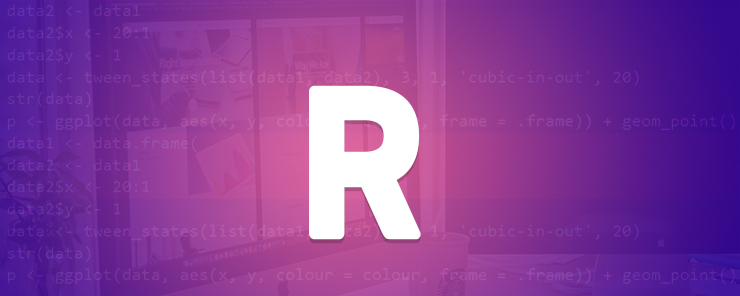To use the R language effectively a good understanding of data types, data structures and how they are operated is important. These objects are the basis of all operations performed in R. For example, a common source of headache is object conversions which can be eliminated with a good understanding of R objects. It is important to note in R that everything is an object and operations are performed as function calls. Data structures in base R can be organized in two ways. The first way of organizing data structures is by their dimensionality which can be 1, 2 or n dimensionality and the second way is by their contents which can be homogeneous or heterogeneous. All the contents in a homogeneous must be of the same type while in a heterogeneous structure contents with different types are allowed.

There are five commonly used data structures in R. An atomic vector is one dimensional and it can only hold homogeneous contents. A matrix is a two dimensional homogeneous structure. An array is an n dimensional homogenous data structure. A list is a one dimensional heterogeneous data structure. A data frame is a two dimensional heterogeneous data structure. It is important to note that R lacks data structures with 0 dimensionality therefore single numbers or strings are represented as a vector having length 1. For any object the str() command provides detailed information about the object.

A vector is the basic data structure in R and it can be represented as a list or an atomic vector. A vector has three properties. The first property is the type which shows what the object is and it can be got using the function typeof(). The second property is the length which shows the number of elements contained in the object and it can be got using the function length(). The third property is attributes which gives additional information and it can be obtained using the function attributes(). An atomic vector requires all the elements to be of the same type and this requirement is relaxed in a list so that a list can have elements of different types. To test if an object is a vector the function is.atomic(x) or is.list(x) is used.

There are four commonly used atomic vectors and they are integer, logical, character and double. Examples of how to create these different vectors are shown below.

`double_vec =  c(2.3, 3.5,4.2,3.1)`

Including an L suffix results in an integer

`integer_vec = c(10L, 5L, 12L, 23L)`

To create a logical vector you can use TRUE, FALSE or T, F elements.

```logical_vec = c(T,T,F,T,F,F)
character_vec = c(“I am learning”, “data structures” , “in R”)```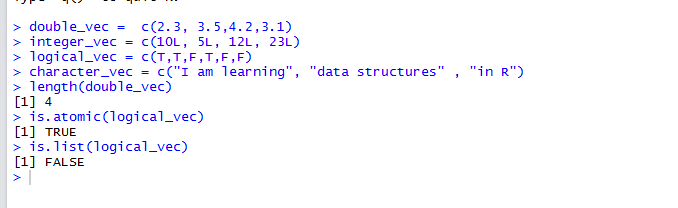Within vector, missing values are supported and they are specified using NA. An attempt at using different types in an atomic vector will result in coercion of elements to most flexible element. Character is the most flexible type while logical is the least flexible type. A double is more flexible than an integer

The second data structure is the list and it differs from a vector because it can hold more than one data type. Another difference is to construct a list you use the list() function instead of c(). The elements of a list can include other lists which makes lists to be referred to as recursive vectors. An example of creating a list is shown below

```my_list = list(0:9, "I am learning", c(T,T,F,T,F), c(2L, 5L,6L))
str(my_list)```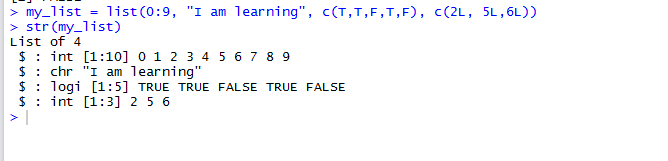Lists are the building blocks of more complex data structures in R. Examples are data frames and linear model objects.

The third data structure is the factor. A factor is a type of a vector having preset values and it is used to hold categorical data. The two important attributes in a factor are class and levels that specify allowable values.

```my_factor = factor(c("Beginner","Intermediate","Advanced"))
my_factor```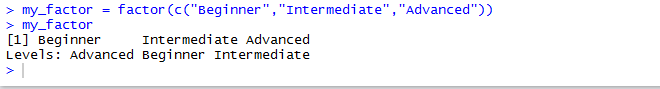The other data structures are matrices and arrays. To create a multidimensional array you include a dim attribute to an atomic vector. When you include a two dimension, it results in a matrix. Matrices are common but arrays are less common. To define a matrix a matrix () function is used while to define an array an array () function is used. An alternative approach is dim (). Examples are shown below

To create a matrix with 4 rows and 2 columns, the command below is used

`my_matrix <- matrix(1:8, ncol = 4, nrow = 2)`

To create an array, the following command below is used

`my_array<- array(1:12, c(2, 3, 2))`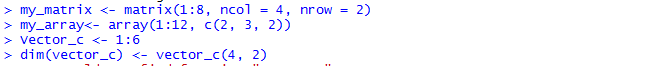Examples of using a dimension attribute to create a matrix is shown below

```vector_c <- 1:8
dim(vector_c) <- c(4, 2)
vector_c```

Creating an array is done as shown below

```dim(vector_c) <- c(2,4)
vector_c```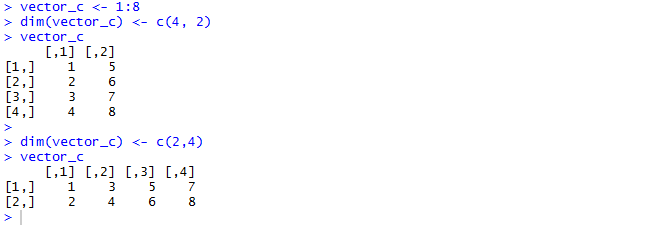The final data structure that will be considered in this article is the data frame which is the most common and it simplifies the process of data analysis. The function data.frame() accepts named vectors and creates a data frame. An example is shown below

`my_dataframe = data.frame(value = c(10,20,30,40), label = c("Beginner","Learner","Intermediate", "Advanced"))`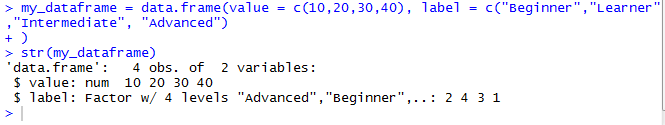The default behavior of data.frame() is to convert strings to factors so when you would prefer your data is not formatted this way, you use stringsAsFactors = FALSE

`my_dataframe = data.frame(value = c(10,20,30,40), label = c("Beginner","Learner","Intermediate", "Advanced"),stringsAsFactors=FALSE)`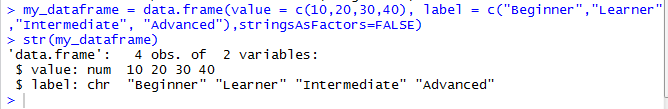To add columns or rows to a data frame, functions cbind() and rbind() are used respectively. Examples are shown below

```mydata = cbind(my_dataframe, data.frame(age = c(21,22, 29,32)))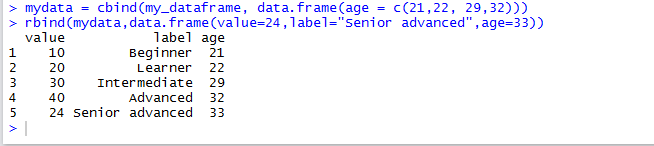Objects allow sub-setting operations which simplifies complex operations. To effectively use sub-setting the concepts listed below need to be understood
• Operators used in sub-setting
• Ways in which you can subset
• Using sub-setting together with assignment
• Ways in which different objects behave

The three sub-setting operators are [, [[ and \$. The [[ operator shares similarities with [ and the only difference is that [[ can be used to get a single value and it enables you get elements from a list. The \$ operator is an abbreviated form of [[.

For an atomic vector positive integers will get you the elements in the positions specified by integers while negative integers will exclude elements. Examples are shown below

```vector_c[c(1,3)]
vector_c[-c(1,3)]```

In this article, we introduced the different data structures that are necessary for data analysis in R. We discussed the properties of each data structure and demonstrated how they are created. We also discussed the properties of data structures. Finally, we discussed how sub-setting is done.